# DRAFT: RLMeta poster 2

Published on 2020-06-13.

This is a work in progress that will change. Like to see it finished? Let me know by sending me an email.

This is a work in progress for a possible second version of the RLMeta poster.

## Evaluation guidelines

From the first poster article:

The smaller it is, the easier it is to understand and therefore extend. The more flexible it is to extend the better. If I make another poster version it would therefore focus on being smaller and more flexible. Since all successive version of RLMeta have been faster than the ones before, performance is also important. But small size, clarity, and flexibility come first.
• Clarity: How does it affect understandability/learnability/readability?
• Size: Lines of code.
• Flexibility: How easy is it to modify RLMeta to be what you need?
• Performance: How fast does it compile?

## Changes

Various changes to improve readability. They do not affect size or performance.

• Rename to make intention more clear.

### Generate labels in semantic actions

One thing that I left in the first version of the poster that still annoyed me was that labels are generated at match time, not at semantic action evaluation time. It will not produce incorrect results. At worst, some labels end up not being used because the counter value captured was in a rule that later failed. But dealing with labels at match time does not make sense. It should really happen at semantic action evaluation time.

Here is what the `Not` rule looks like in the first version of the poster:

```Not = ast:x #:a #:b -> { "I('BACKTRACK', " b ")\n"
x
"I('COMMIT', " a ")\n"
"LABEL(" a ")\n"
"I('FAIL', 'no match expected')\n"
"LABEL(" b ")\n"                   }
```

Here is what the `Not` rule looks like after the change:

```Not = ast:x -> label():a -> label():b
-> { "I('BACKTRACK', " b ")\n"
x
"I('COMMIT', " a ")\n"
"LABEL(" a ")\n"
"I('FAIL', 'no match expected')\n"
"LABEL(" b ")\n"                   }

```

This change puts label generation where it belongs, in semantic actions, and thus makes the implementation more clear. The VM is no longer concerned with labels. It is only concerned with matching. It does make semantic actions a bit more complicated, but the bind syntax is familiar from match expressions and an action being a sequence of things should be familiar as well.

This change required a bit of rework how semantic actions work. Previously only one expression was allowed. Now multiple expressions are allowed. The result of expressions can also be bound to names which subsequent expressions can refer to. Furthermore, there are now also runtime variables that are set with bindings. `label` is a built in runtime function that generates increasing integers starting at 0.

The implementation of this change also increases the flexibility of RLMeta. For example, it is now possible to write a semantic action that generates code in different sections like this:

1. example_buffers.rlmeta
```ExampleBuffers {
"# BODY\n"
x            }
ast      = [%:x]  -> x
Program  = ast*
Function = .:name -> header({ "def " name "\n" })
-> { name "()\n" }
}
```

`Buffer` is a specialised list that appends an element when called as a function:

1. example_buffers.py
```class Buffer(list):

def __call__(self, arg):
self.append(arg)
```

Here is an example AST representing a program:

```[
['Program',
['Function', 'foo'],
['Function', 'bar']
]
]
```

When the `program` rule is run on the example input, the following is output:

```# HEADER
def foo
def bar
# BODY
foo()
bar()

```

This type of thing is useful for example when generating C functions where definitions need to go in "header" and declarations in "body".

In summary, this change is as follows:

• Label syntax (`#`) in parser is removed
• Actions can have multiple expressions
• Expressions can be bound to names
• A default `label` function to generate labels
• Names in semantic actions refer to matches or results bound in semantic actions
• New syntax `#name` is used in semantic actions to refer to "native" names

The complete diff for this change can be found on GitHub.

The increased clarity and flexibility come with a price. The size increases and the performance drops.

```\$ wc -l parser.rlmeta codegenerator.rlmeta support.py compile.sh
56 parser.rlmeta        (+5)
68 codegenerator.rlmeta (+7)
243 support.py           (+27)
54 compile.sh           (0)
421 total                (+39)
```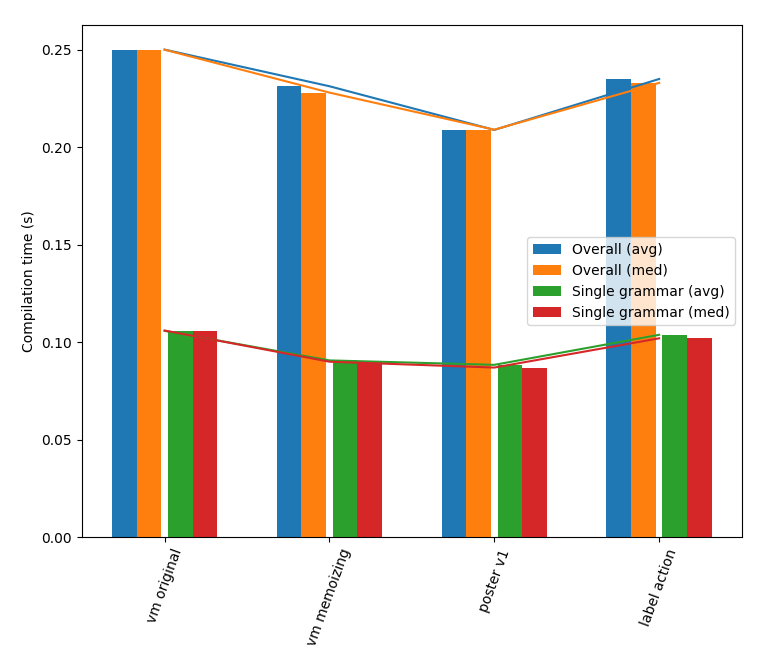Performance measurements.

The parser and the code generator are mostly the same. The greatest addition is in the support library. Which is expected when semantic action evaluation becomes more complex. The drop in performance is likely due to more function calls when evaluating semantic actions. Even though size and performance got worse, I believe the clarity and flexibility gain is worth it.

```astItems =
| ast:x astItem*:xs  -> { x xs   }
|                    -> {        }
astItem  = ast:x       -> { ", " x }
```
```astItems = ast*:xs     -> #join(xs ", ")
```

The complete diff for this change can be found on GitHub.

```\$ wc -l parser.rlmeta codegenerator.rlmeta support.py compile.sh
56 parser.rlmeta        (0)
65 codegenerator.rlmeta (-3)
243 support.py           (0)
54 compile.sh           (0)
418 total                (-3)
```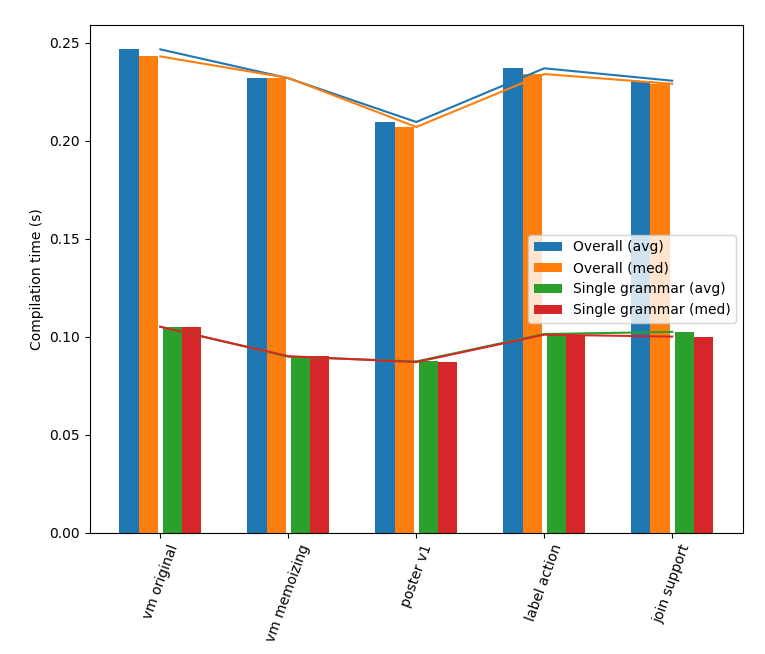Performance measurements.

### Disallow semantic actions in the middle

```\$ echo "Grammar { rule = . ->[] . }" | python rlmeta.py
class Grammar(Grammar):

def assemble(self, I, LABEL):
LABEL('rule')
I('PUSH_SCOPE')
I('MATCH_ANY')
I('ACTION', lambda scope: concat([]))
I('MATCH_ANY')
I('POP_SCOPE')
I('RETURN')
```
```\$ echo "Grammar { rule = . ->[] . }" | python rlmeta.py
ERROR: expected '}'
POSITION: 24
STREAM:
Grammar { rule = . ->[] <ERROR POSITION>. }
```

The complete diff for this change can be found on GitHub.

```\$ wc -l parser.rlmeta codegenerator.rlmeta support.py compile.sh
58 parser.rlmeta        (+2)
65 codegenerator.rlmeta (0)
243 support.py           (0)
54 compile.sh           (0)
420 total                (+2)
```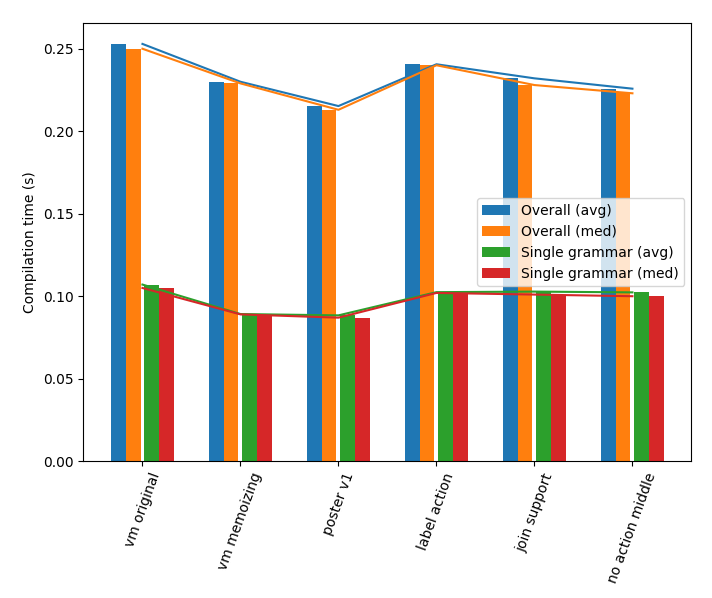Performance measurements.

### Allow indent prefix to be changed

```def indent(text):
return join(join(["    ", line]) for line in text.splitlines(True))
```
```def indent(text, prefix="    "):
return "".join(prefix + line for line in text.splitlines(True))

```

The complete diff for this change can be found on GitHub.

```\$ wc -l parser.rlmeta codegenerator.rlmeta support.py compile.sh
58 parser.rlmeta        (0)
66 codegenerator.rlmeta (+1)
244 support.py           (+1)
54 compile.sh           (0)
422 total                (+2)
```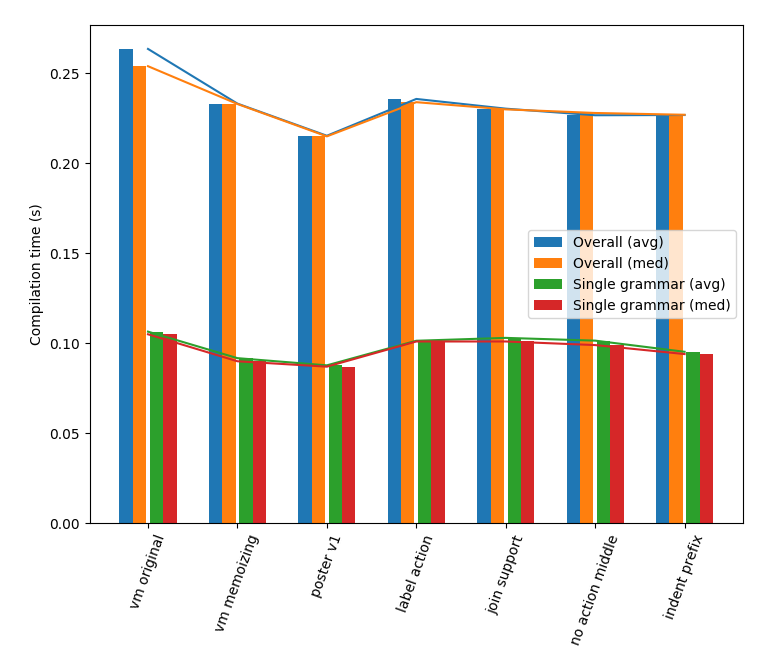Performance measurements.

Better flexibility at marginal cost. A little slower, but fixed by optimizing `indent` a little.

### Runtime vars outside VM

The complete diff for this change can be found on GitHub.

```\$ wc -l parser.rlmeta codegenerator.rlmeta support.py compile.sh
58 parser.rlmeta        (0)
66 codegenerator.rlmeta (0)
241 support.py           (-3)
54 compile.sh           (0)
419 total                (-3)
```

Should not and does not affect performance.

### Remove dependency on Bash

The complete diff for this change can be found on GitHub.

```def compile_grammar(grammar):
return CodeGenerator().run(
"ast",
[Parser().run("grammar", grammar)]
)

if __name__ == "__main__":
import sys
import pprint
if path == "-":
with open(path) as f:
args = sys.argv[1:] or ["--compile", "-"]
while args:
command = args.pop(0)
if command == "--support":
sys.stdout.write(SUPPORT)
elif command == "--copy":
elif command == "--embed":
sys.stdout.write("{} = {}\n".format(
args.pop(0),
))
elif command == "--compile":
try:
except MatchError as e:
stream = e.stream
for pos in e.pos[:-1]:
stream = stream[pos]
pos = e.pos[-1]
MARKER = "\033[0;31m<ERROR POSITION>\033[0m"
if isinstance(stream, basestring):
stream_string = stream[:pos] + MARKER + stream[pos:]
else:
stream_string = pprint.pformat(stream)
sys.exit("ERROR: {}\nPOSITION: {}\nSTREAM:\n{}".format(
e.message,
pos,
indent(stream_string)
))
else:
sys.exit("ERROR: Unknown command '{}'".format(command))
```
```python rlmeta.py \
--embed SUPPORT support.py \
--support \
--compile parser.rlmeta \
--compile codegenerator.rlmeta \
--copy main.py
```
```\$ wc -l parser.rlmeta codegenerator.rlmeta support.py main.py
58 parser.rlmeta        (0)
66 codegenerator.rlmeta (0)
241 support.py           (0)
46 main.py              (+46)
411 total                (-8)
```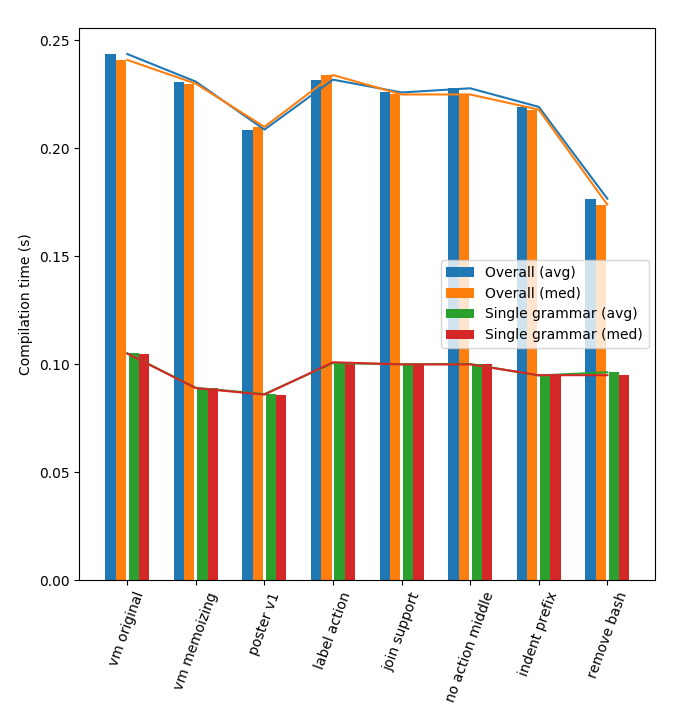Performance measurements.

The `rlmeta.py` file that is created is almost identical. So compiling a single grammar takes the same amount of time. However, compiling rlmeta, putting together `rlmeta.py`, that was previously done in Bash and now done in Python, has gotten faster.

### Possible next changes

• Substream matching should return substream as action
• Macro language for creating VMs in Python
• `run` -> `match` rename of grammar method
• What should an empty ["And"] return? Undefined or default `None`?
• `?` operator should return empty list (in case of no match) or list with one item (in case of match).
• Add one more pass in between parser and codegen that generates VM-instrucionts
• VM-instructions will be easier to read
• Possible for peephole optimizations before generating Python code

#### Only one match instruction

Diff:

```diff --git a/writing/rlmeta-poster2/codegenerator.rlmeta b/writing/rlmeta-poster2/codegenerator.rlmeta
index 205b89e..174e336 100644
--- a/writing/rlmeta-poster2/codegenerator.rlmeta
+++ b/writing/rlmeta-poster2/codegenerator.rlmeta
@@ -40,9 +40,9 @@ CodeGenerator {
Label          =                 -> { "I('LABEL')\n"                       }
SemanticAction = ast:x           -> { "I('ACTION', lambda scope: " x ")\n" }
MatchRule      = py:x            -> { "I('CALL', " x ")\n"                 }
-  MatchRange     = py:x py:y       -> { "I('MATCH_RANGE', " x ", " y ")\n"   }
-  MatchObject    = py:x            -> { "I('MATCH_OBJECT', " x ")\n"         }
-  MatchAny       =                 -> { "I('MATCH_ANY')\n"                   }
+  MatchRange     = py:x py:y       -> { "I('MATCH', *range(" x ", " y "))\n" }
+  MatchObject    = py:x            -> { "I('MATCH', *exact(" x "))\n"        }
+  MatchAny       =                 -> { "I('MATCH', 'any', any)\n"           }
MatchList      = ast:x           -> { "I('PUSH_STREAM')\n"
x
"I('POP_STREAM')\n"                  }
diff --git a/writing/rlmeta-poster2/support.py b/writing/rlmeta-poster2/support.py
index 97100ff..3b5d69e 100644
--- a/writing/rlmeta-poster2/support.py
+++ b/writing/rlmeta-poster2/support.py
@@ -21,6 +21,18 @@ def vm(instructions, labels, start_rule, stream):
))
pc += 1
continue
+        elif name == "MATCH":
+            if pos >= len(stream):
+                fail_message = ("expected {}, found EOF", arg1)
+            else:
+                value = stream[pos]
+                if arg2(value):
+                    action = SemanticAction(value)
+                    pos += 1
+                    pc += 1
+                    continue
+                else:
+                    fail_message = ("expected {}, found {!r}", arg1, value)
elif name == "CALL":
key = (arg1, tuple([x for x in stream_pos_stack]+[pos]))
if key in memo:
@@ -40,14 +52,6 @@ def vm(instructions, labels, start_rule, stream):
scope = scope_stack.pop()
pc += 1
continue
-        elif name == "MATCH_OBJECT":
-            if pos >= len(stream) or stream[pos] != arg1:
-                fail_message = ("expected {!r}", arg1)
-            else:
-                action = SemanticAction(arg1)
-                pos += 1
-                pc += 1
-                continue
elif name == "COMMIT":
call_backtrack_stack.pop()
pc = labels[arg1]
@@ -70,14 +74,6 @@ def vm(instructions, labels, start_rule, stream):
action = SemanticAction(scope, arg1)
pc += 1
continue
-        elif name == "MATCH_RANGE":
-            if pos >= len(stream) or not (arg1 <= stream[pos] <= arg2):
-                fail_message = ("expected range {!r}-{!r}", arg1, arg2)
-            else:
-                action = SemanticAction(stream[pos])
-                pos += 1
-                pc += 1
-                continue
elif name == "LIST_START":
scope_stack.append(scope)
scope = []
@@ -88,14 +84,6 @@ def vm(instructions, labels, start_rule, stream):
scope = scope_stack.pop()
pc += 1
continue
-        elif name == "MATCH_ANY":
-            if pos >= len(stream):
-                fail_message = ("expected any",)
-            else:
-                action = SemanticAction(stream[pos])
-                pos += 1
-                pc += 1
-                continue
elif name == "PUSH_STREAM":
if pos >= len(stream) or not isinstance(stream[pos], list):
fail_message = ("expected list",)
@@ -214,3 +202,12 @@ def join(items):

def indent(text):
return join(join(["    ", line]) for line in text.splitlines(True))
+
+def any(x):
+    return True
+
+def exact(value):
+    return "object {!r}".format(value), lambda x: x == value
+
+def range(a, b):
+    return "range {!r}-{!r}".format(a, b), lambda x: a <= x <= b
```

The implementation is a bit more flexible and reduces the number of VM instructions.

Line count:

```\$ wc -l parser.rlmeta codegenerator.rlmeta support.py compile.sh
51 parser.rlmeta
61 codegenerator.rlmeta
213 support.py
54 compile.sh
379 total
```

Only 3 lines shorter. The helper functions could be inlined in code generator. But that would make it harder to read.

Performance: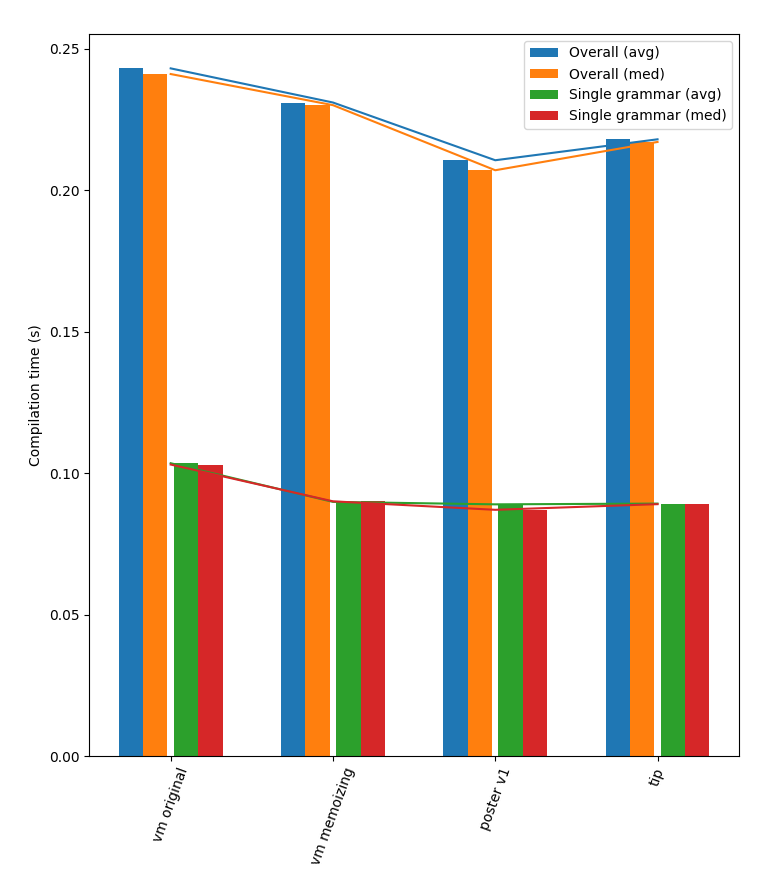Performance measurements.

A little slower.

Summary:

I will hold off on this change. It became slower and not much smaller. If the flexibility is needed, it might be worth it.

## Code listings for RLMeta

The code here is exactly the same as on the poster. The `meta_compile.sh` script is not shown on the poster since it is not strictly part of the implementation. It is more of a developer tool.

### parser.rlmeta

1. parser.rlmeta
```Parser {
grammar =
| name:x space '{' rule*:ys space '}'     -> ["Grammar" x ~ys]
rule =
| name:x space '=' choice:y               -> ["Rule" x y]
choice =
| (space '|')?
sequence:x (space '|' sequence)*:xs     -> ["Or" x ~xs]
sequence =
| expr*:xs maybeAction:ys                 -> ["Scope" ["And" ~xs ~ys]]
expr =
| expr1:x space ':' name:y                -> ["Bind" y x]
| expr1
expr1 =
| expr2:x space '*'                       -> ["Star" x]
| expr2:x space '?'                       -> ["Or" x ["And"]]
| space '!' expr2:x                       -> ["Not" x]
| space '%'                               -> ["MatchCallRule"]
| expr2
expr2 =
| name:x !(space '=')                     -> ["MatchRule" x]
| space char:x '-' char:y                 -> ["MatchRange" x y]
| space '\'' (!'\'' matchChar)*:xs '\''   -> ["And" ~xs]
| space '.'                               -> ["MatchAny"]
| space '(' choice:x space ')'            -> x
| space '[' expr*:xs space ']'            -> ["MatchList" ["And" ~xs]]
matchChar =
| innerChar:x                             -> ["MatchObject" x]
maybeAction =
| actionExpr:x actionExpr*:xs             -> [["Action" ~x ~~xs]]
|                                         -> []
actionExpr =
| space '->' hostExpr:x
(space ':' name | -> ""):y              -> [y  x]
hostExpr =
| space string:x                          -> ["String" x]
| space '[' hostListItem*:xs space ']'    -> ["List" ~xs]
| space '{' formatExpr*:xs space '}'      -> ["Format" ~xs]
| var:x space '(' hostExpr*:ys space ')'  -> ["Call" x ~ys]
| var:x
hostListItem =
| space '~'*:ys hostExpr:x                -> ["ListItem" #len(ys) x]
formatExpr =
| space '>' formatExpr*:xs space '<'      -> ["Indent" ["Format" ~xs]]
| hostExpr
var =
| space '#' name:x                        -> ["Native" x]
| name:x !(space '=')                     -> ["Lookup" x]
string    = '"'  (!'"'  innerChar)*:xs '"'  -> { xs }
char      = '\''  !'\'' innerChar  :x  '\'' -> x
innerChar = '\\' escape | .
escape    = '\\' -> "\\" | '\'' -> "'"
| '"'  -> "\"" | 'n'  -> "\n"
name      = space nameStart:x nameChar*:xs  -> { x xs }
nameStart = 'a'-'z' | 'A'-'Z'
nameChar  = 'a'-'z' | 'A'-'Z' | '0'-'9'
space     = (' ' | '\n')*
}
```

### codegenerator.rlmeta

1. codegenerator.rlmeta
```CodeGenerator {
ast           = [%:x]        -> x
Grammar       = .:x ast*:ys  -> { "class " x "(Grammar):\n\n" >
"def assemble(self, I, LABEL):\n" >
ys
<
<                                    }
Rule          = py:x ast:y   -> { "LABEL(" x ")\n"
y
"I('RETURN')\n"                      }
Or            =
| ast:x Or:y               -> label():a -> label():b
-> { "I('BACKTRACK', " a ")\n"
x
"I('COMMIT', " b ")\n"
"LABEL(" a ")\n"
y
"LABEL(" b ")\n"                     }
| ast
Scope         = ast:x        -> { "I('PUSH_SCOPE')\n"
x
"I('POP_SCOPE')\n"                   }
And           = ast*
Bind          = py:x ast:y   -> { y
"I('BIND', " x ")\n"                 }
Star          = ast:x        -> label():a -> label():b
-> { "I('LIST_START')\n"
"LABEL(" a ")\n"
"I('BACKTRACK', " b ")\n"
x
"I('LIST_APPEND')\n"
"I('COMMIT', " a ")\n"
"LABEL(" b ")\n"
"I('LIST_END')\n"                    }
Not           = ast:x        -> label():a -> label():b
-> { "I('BACKTRACK', " b ")\n"
x
"I('COMMIT', " a ")\n"
"LABEL(" a ")\n"
"I('FAIL', 'no match expected')\n"
"LABEL(" b ")\n"                     }
MatchCallRule =              -> { "I('MATCH_CALL_RULE')\n"             }
MatchRule     = py:x         -> { "I('CALL', " x ")\n"                 }
MatchRange    = py:x py:y    -> { "I('MATCH_RANGE', " x ", " y ")\n"   }
MatchAny      =              -> { "I('MATCH_ANY')\n"                   }
MatchList     = ast:x        -> { "I('PUSH_STREAM')\n"
x
"I('POP_STREAM')\n"                  }
MatchObject   = py:x         -> { "I('MATCH_OBJECT', " x ")\n"         }
Action        = actionExpr:x -> { "I('ACTION', lambda scope: " x ")\n" }
actionExpr    =
| py:x ast:y actionExpr:z  -> { "scope.bind("
x ", " y ", lambda: " z ")"          }
| .    ast
String        = py
List          = asts:x       -> { "concat([" x "])"                    }
ListItem      = py:x ast:y   -> { "splice(" x ", " y ")"               }
Format        = asts:x       -> { "join([" x "])"                      }
Indent        = ast:x        -> { "indent(" x ", "
"scope.lookup('indentprefix'))"      }
Call          = ast:x asts:y -> { x "(" y ")"                          }
Native        = .
Lookup        = py:x         -> { "scope.lookup(" x ")"                }
asts          = ast*:xs      -> #join(xs ", ")
py            = .:x          -> #repr(x)
}
```

### support.py

1. support.py
```def vm(instructions, labels, start_rule, stream, runtime):
action = SemanticAction(None)
pc = labels[start_rule]
call_backtrack_stack = []
stream, pos, stream_pos_stack = (stream, 0, [])
scope, scope_stack = (None, [])
fail_message = None
latest_fail_message, latest_fail_pos = (None, tuple())
memo = {}
while True:
name, arg1, arg2 = instructions[pc]
if name == "PUSH_SCOPE":
scope_stack.append(scope)
scope = {}
pc += 1
continue
elif name == "BACKTRACK":
call_backtrack_stack.append((
labels[arg1], pos, len(stream_pos_stack), len(scope_stack)
))
pc += 1
continue
elif name == "CALL":
key = (arg1, tuple([x for x in stream_pos_stack]+[pos]))
if key in memo:
if memo[key] is None:
fail_message = memo[key]
else:
action, stream_pos_stack = memo[key]
stream_pos_stack = stream_pos_stack[:]
stream, pos = stream_pos_stack.pop()
pc += 1
continue
else:
call_backtrack_stack.append((pc+1, key))
pc = labels[arg1]
continue
elif name == "POP_SCOPE":
scope = scope_stack.pop()
pc += 1
continue
elif name == "MATCH_OBJECT":
if pos >= len(stream) or stream[pos] != arg1:
fail_message = ("expected {!r}", arg1)
else:
action = SemanticAction(arg1)
pos += 1
pc += 1
continue
elif name == "COMMIT":
call_backtrack_stack.pop()
pc = labels[arg1]
continue
elif name == "RETURN":
if len(call_backtrack_stack) == 0:
return action.eval()
pc, key = call_backtrack_stack.pop()
memo[key] = (action, stream_pos_stack+[(stream, pos)])
continue
elif name == "LIST_APPEND":
scope.append(action)
pc += 1
continue
elif name == "BIND":
scope[arg1] = action
pc += 1
continue
elif name == "ACTION":
action = SemanticAction(Scope(scope, runtime), arg1)
pc += 1
continue
elif name == "MATCH_RANGE":
if pos >= len(stream) or not (arg1 <= stream[pos] <= arg2):
fail_message = ("expected range {!r}-{!r}", arg1, arg2)
else:
action = SemanticAction(stream[pos])
pos += 1
pc += 1
continue
elif name == "LIST_START":
scope_stack.append(scope)
scope = []
pc += 1
continue
elif name == "LIST_END":
action = SemanticAction(scope, lambda xs: [x.eval() for x in xs])
scope = scope_stack.pop()
pc += 1
continue
elif name == "MATCH_ANY":
if pos >= len(stream):
fail_message = ("expected any",)
else:
action = SemanticAction(stream[pos])
pos += 1
pc += 1
continue
elif name == "PUSH_STREAM":
if pos >= len(stream) or not isinstance(stream[pos], list):
fail_message = ("expected list",)
else:
stream_pos_stack.append((stream, pos))
stream = stream[pos]
pos = 0
pc += 1
continue
elif name == "POP_STREAM":
if pos < len(stream):
fail_message = ("expected end of list",)
else:
stream, pos = stream_pos_stack.pop()
pos += 1
pc += 1
continue
elif name == "MATCH_CALL_RULE":
if pos >= len(stream):
fail_message = ("expected any",)
else:
fn_name = str(stream[pos])
key = (fn_name, tuple([x for x in stream_pos_stack]+[pos]))
if key in memo:
if memo[key] is None:
fail_message = memo[key]
else:
action, stream_pos_stack = memo[key]
stream_pos_stack = stream_pos_stack[:]
stream, pos = stream_pos_stack.pop()
pc += 1
continue
else:
call_backtrack_stack.append((pc+1, key))
pc = labels[fn_name]
pos += 1
continue
elif name == "FAIL":
fail_message = (arg1,)
else:
raise Exception("unknown instruction {}".format(name))
fail_pos = tuple([x for x in stream_pos_stack]+[pos])
if fail_pos >= latest_fail_pos:
latest_fail_message = fail_message
latest_fail_pos = fail_pos
call_backtrack_entry = tuple()
while call_backtrack_stack:
call_backtrack_entry = call_backtrack_stack.pop()
if len(call_backtrack_entry) == 4:
break
else:
_, key = call_backtrack_entry
memo[key] = (None, fail_message)
if len(call_backtrack_entry) != 4:
raise MatchError(
latest_fail_message.format(*latest_fail_message[1:]),
latest_fail_pos,
stream_pos_stack if stream_pos_stack else stream
)
(pc, pos, stream_stack_len, scope_stack_len) = call_backtrack_entry
if len(stream_pos_stack) > stream_stack_len:
stream = stream_pos_stack[stream_stack_len]
stream_pos_stack = stream_pos_stack[:stream_stack_len]
if len(scope_stack) > scope_stack_len:
scope = scope_stack[scope_stack_len]
scope_stack = scope_stack[:scope_stack_len]

class Scope(object):

def __init__(self, match, runtime):
self.match = match
self.runtime = runtime

def bind(self, name, value, continuation):
old = self.runtime.get(name, None)
self.runtime[name] = value
try:
return continuation()
finally:
self.runtime[name] = old

def lookup(self, name):
if name in self.match:
return self.match[name].eval()
else:
return self.runtime.get(name, None)

class SemanticAction(object):

def __init__(self, value, fn=lambda value: value):
self.value = value
self.fn = fn

def eval(self):
return self.fn(self.value)

class MatchError(Exception):

def __init__(self, message, pos, stream):
Exception.__init__(self)
self.message = message
self.pos = pos
self.stream = stream

class Grammar(object):

def __init__(self):
self.instructions = instructions = []
self.labels = labels = {}
def I(name, arg1=None, arg2=None):
instructions.append((name, arg1, arg2))
def LABEL(name):
labels[name] = len(instructions)
self.assemble(I, LABEL)

def run(self, rule_name, stream):
self.label_counter = 0
return vm(self.instructions, self.labels, rule_name, stream, {
"label": self.next_label,
"indentprefix": "    ",
})

def next_label(self):
result = self.label_counter
self.label_counter += 1
return result

def splice(depth, item):
if depth == 0:
return [item]
else:
return concat([splice(depth-1, subitem) for subitem in item])

def concat(lists):
return [x for xs in lists for x in xs]

def join(items, delimiter=""):
return delimiter.join(
join(item, delimiter) if isinstance(item, list) else str(item)
for item in items
)

def indent(text, prefix="    "):
return "".join(prefix+line for line in text.splitlines(True))
```

### main.py

1. main.py
```def compile_grammar(grammar):
return CodeGenerator().run(
"ast",
[Parser().run("grammar", grammar)]
)

if __name__ == "__main__":
import sys
import pprint
if path == "-":
with open(path) as f:
args = sys.argv[1:] or ["--compile", "-"]
while args:
command = args.pop(0)
if command == "--support":
sys.stdout.write(SUPPORT)
elif command == "--copy":
elif command == "--embed":
sys.stdout.write("{} = {}\n".format(
args.pop(0),
))
elif command == "--compile":
try:
except MatchError as e:
stream = e.stream
for pos in e.pos[:-1]:
stream = stream[pos]
pos = e.pos[-1]
MARKER = "\033[0;31m<ERROR POSITION>\033[0m"
if isinstance(stream, basestring):
stream_string = stream[:pos] + MARKER + stream[pos:]
else:
stream_string = pprint.pformat(stream)
sys.exit("ERROR: {}\nPOSITION: {}\nSTREAM:\n{}".format(
e.message,
pos,
indent(stream_string)
))
else:
sys.exit("ERROR: Unknown command '{}'".format(command))
```

### make.py

1. make.py
```#!/usr/bin/env python

import os
import subprocess
import sys

def make_next_version():
intermediate_compilers = meta_compile_rlmeta()
final_compiler = intermediate_compilers.pop(-1)
test(final_compiler)
mv(final_compiler, "rlmeta.py")
for compiler in intermediate_compilers:
rm(compiler)
log("OK!")

def meta_compile_rlmeta():
compiler = "rlmeta.py"
intermediate_compilers = []
for i in range(4):
next_compiler = "rlmeta{}.py".format(i+1)
log("Compiling {} -> {}".format(compiler, next_compiler))
next_content = compile_rlmeta(compiler)
write(next_compiler, next_content)
intermediate_compilers.append(next_compiler)
if next_content == content:
return intermediate_compilers
compiler = next_compiler
content = next_content
fail("Unable to produce metacompiler.")

def compile_rlmeta(rlmeta):
return run_rlmeta(rlmeta, [
"--embed", "SUPPORT", "support.py",
"--support",
"--compile", "parser.rlmeta",
"--compile", "codegenerator.rlmeta",
"--copy", "main.py",
])

def test(rlmeta):
log("Test: Has its own support library")
log("Test: Disallow semantic action in the middle")
run_rlmeta(rlmeta, [], "Grammar { x = . -> [] . }", expect_failure=True)

def run_rlmeta(rlmeta, args, stdin="", expect_failure=False):
process = subprocess.Popen(
["python", rlmeta]+args,
stdin=subprocess.PIPE,
stdout=subprocess.PIPE
)
stdout, _ = process.communicate(stdin)
if expect_failure:
if process.returncode == 0:
fail("Expected failure")
else:
if process.returncode != 0:
fail("Expected success")
return stdout

def mv(src, dest):
log("Move {} -> {}".format(src, dest))
os.remove(dest)
os.rename(src, dest)

def rm(path):
log("Delete {}".format(path))
os.remove(path)

with open(path) as f:

def write(path, content):
with open(path, "w") as f:
return f.write(content)

def log(message):
sys.stdout.write("\033[0;33m{}\033[0m\n".format(message))

def fail(message):
sys.exit("\033[0;31mERROR: {}\033[0m".format(message))

if __name__ == "__main__":
if sys.argv[1:] == ["compile"]:
sys.stdout.write(compile_rlmeta("rlmeta.py"))
else:
make_next_version()
```

Site proudly generated by Hakyll.